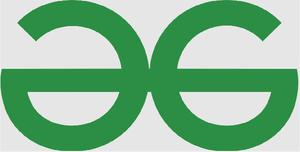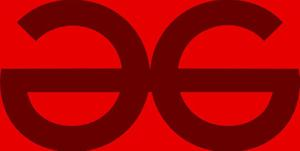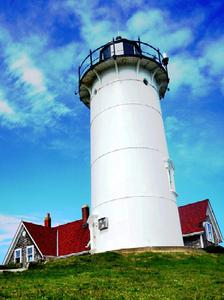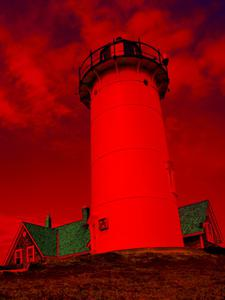# How to Convert RGB Image to YIQ Image using MATLAB?

• Last Updated : 08 Mar, 2022

Image Processing in MATLAB use functions from the Image Processing Toolbox. This toolbox generally represents colors as RGB numeric values. Different models also exist for representing colors numerically. The official term for these models is “color spaces” and is coined from the definition of a vector space as these can be mapped in a 2D,3D, or 4D coordinate system.

The most important advantage of having different color spaces and toggling between them is that they make certain calculations more convenient depending on the type of result the user desires. Mathematical transformations are used to interchange among the color spaces. The YIQ color space is used in televisions in the United States. This color space separates grayscale information from color data, so the same signal can be used for both color and black and white television sets thereby increasing usability.

In this article, we will be seeing how to convert an RGB Image to YIQ using MATLAB.

### Approach:

• Use the rgb2ntsc() command to apply the transformations and convert to YIQ space.
• Show both the Images together for comparison purposes.

Example 1:

## Matlab

 `% Matlab Code to convert an RGB``% Image to Binary image reading image``I = imread(``'GFG.jpeg'``);` `% Creating figure window for input image``% Displaying the input image``imshow(I);` `% Converting the image from rgb to``% binary using thresholding``J = rgb2ntsc(I);` `% Creating figure window for the output image``% Displaying the output image``imshow(J);`

Output:Figure 1: Input imageFigure 2: Output image

Consider another example with MATLAB’s inbuilt image of a lighthouse.

Example 2:

## Matlab

 `% Matlab Code to convert an RGB Image``% to Binary image reading image``I = imread(``'lighthouse.png'``);` `% Creating figure window for input image``% Displaying the input image``imshow(I);` `% Converting the image from rgb to``% binary using thresholding``J = rgb2ntsc(I);` `% Creating figure window for the output image``% Displaying the output image``imshow(J);`

Output :Figure 3: Input imageFigure 4: Output image

Code Explanation: